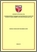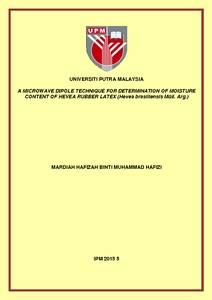# A microwave dipole technique for determination of moisture content of hevea rubber latex (Hevea brasiliensis Mull. Arg.)

## Citation

Muhammad Hafizi, Mardiah Hafizah (2015) A microwave dipole technique for determination of moisture content of hevea rubber latex (Hevea brasiliensis Mull. Arg.). Masters thesis, Universiti Putra Malaysia.

## Abstract

This thesis describes a microwave reflection technique utilising a dipole sensor for determination of moisture content (MC) in hevea rubber latex (Hevea Brasiliensis). In this study the dipole sensor is used to predict the MC in hevea rubber latex by constructing a calibration equation and validating by comparing with standard method which is the over drying method. A simulation study was done using Finite Element Method (FEM) and was analyzed with measured data. The design and simulation of the dipole were carried out using Finite Element Method (FEM) in conjunction with a COMSOL Multiphysics version 3.5 software. The measurement setup for reflection measurement consists of a dipole sensor connected to a Professional Network Analyzer N5230A (PNA). All calibrations and measurements were done in the frequency range between 0.1 GHz and 4 GHz. The comparisons between measured and calculated results were carried out for unloaded sensor as well as the sensor loaded in water and hevea rubber latex sample. Complex permittivity values required as inputs to FEM modelling were obtained using an open ended coaxial probe. Relationships between dielectric constant (ε′), loss factor (ε˝), loss tangent (tan δ) percentages of MC were obtained from the study. Calibration equations relating MC to the ε′, ε˝, tan δ and magnitude of reflection coefficient (|Γ|) at various frequencies were analysed. The calibration equation based on (ε′) at 3.6 GHz was found to be the most accurate in the determination of MC with mean relative error 0.02 when compared to actual MC obtained from standard oven drying method. Calibration equations have also been obtained to predict the amount of MC from the measured |Γ|. The accuracy of the equations was determined by comparing the predicted and actual MC obtained using the calibration equation and oven drying method, respectively. The lowest mean relative error of the calibration equation for |Γ| was found to be 0.048 at 1.5 GHz. The calibration equation for reflection measurement at 1.5 GHz was established to be most accurate with mean relative error 0.048.Preview
PDF
IPM 2015 5IR.pdfView Item